# Generate 3 Unique Random numbers in Microsoft Excel

If you want to generate a list of unique random numbers then you can use RANDBETWEEN functions & VBA RND. In this article, we will generate 3 unique numbers from the provided list.

You can find original question here

Question: I want a macro to generate list of 3 random numbers from a list of numbers saved in column A. There is a condition that VBA code needs to be taken care of. The condition is there are 3 numbers that exist in column B, hence macro needs to create a list of numbers that have not occurred once in column B. In this way, we can have a list of unique numbers in column B & C.

Following is the snapshot of example that we have: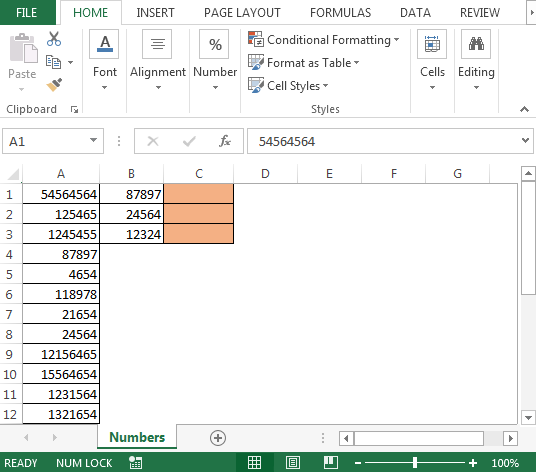To get the list of unique random numbers; we need to follow the below steps to launch VB editor

• Click on Developer tab
• From Code group select Visual Basic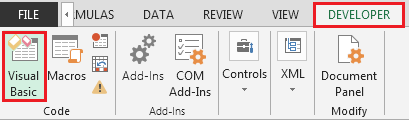• Copy the below code in the standard module

```Sub RandomNumbers()
Dim ws As Worksheet
Dim ar As Variant
Dim RandomNum As Long
Dim i As Integer
Dim myVal As Long
Randomize
Set ws = ThisWorkbook.Sheets("Numbers")
With ws
ar = .Range("A" & Rows.Count).End(xlUp).Row
.Range("C1:C3").ClearContents
For i = 1 To 3
Do
RandomNum = Int((1 - ar + 1) * Rnd + ar)
myVal = .Range("A" & RandomNum).Value
Loop Until Range("B1:C24").Find(what:=myVal, lookat:=xlWhole) Is Nothing
.Range("C" & i).Value = myVal
Next i
End With
End Sub
```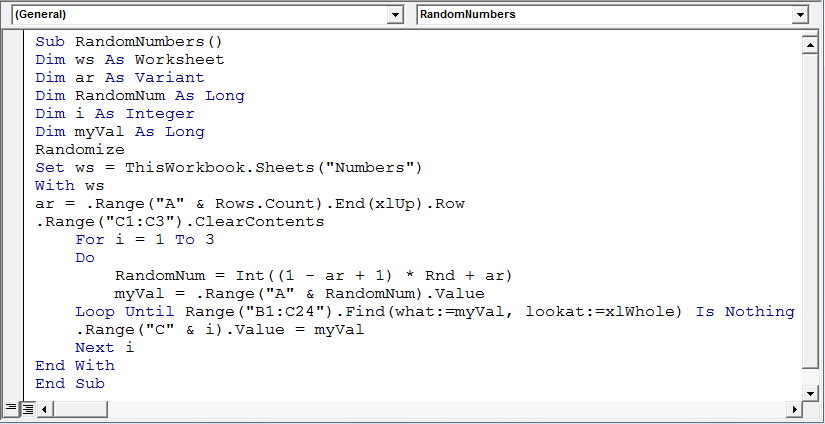• To run the macro, we can press F5 key from keyboard if you are active in Visual Basic Editor’s screen
• Another way to run the macro is using “ALT + F8” key; select the macro & click on Run button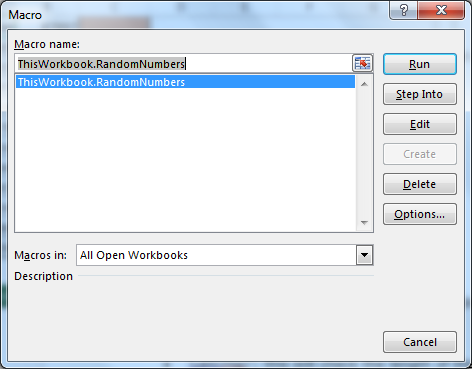• As we run the VBA code, the unique list of numbers will be automatically generated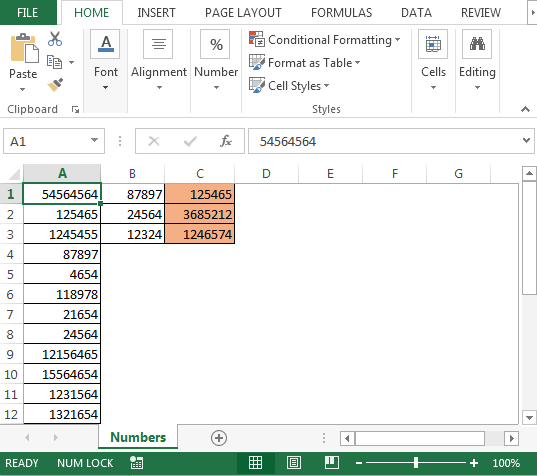Code Explanation:

In the above code, we will use RND & INT function along with For loop & Do loop to get the result.

• We declare Dim ws as Worksheet; the purpose of declaring ws as Worksheet is we only want the code to run on a specific worksheet i.e. Numbers in our example
• Dim RandomNum As Long: this will save the unique numbers
• Set ws = ThisWorkbook.Sheets("Numbers"): this line will ensure that the code runs on Numbers sheet only
• The next line of code will use With statement
• ar = .Range("A" & Rows.Count).End(xlUp).Row: this line will save the last cell in column A (24 in our example)
• .Range("C1:C3").ClearContents: this line will erase any existing content in range C1:C3
• Next, we will run For loop starting from 1 to 3 times with Do Loop to generate list of random numbers & checking no duplicates in existing range i.e. column B

Conclusion: We can generate list of distinct random numbers from a list in Microsoft excel with small tweaking in the above VBA code.